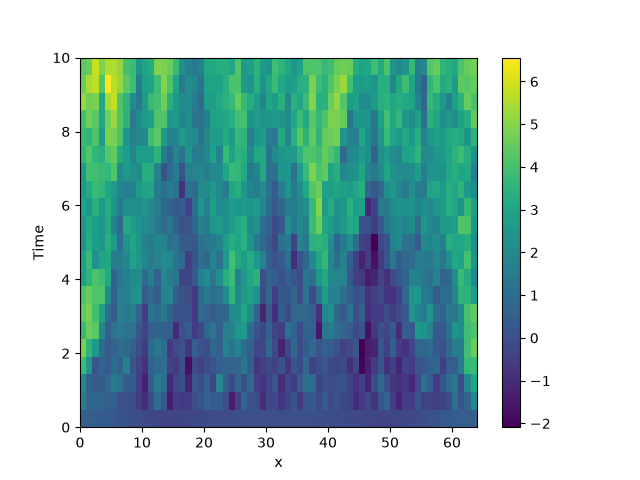# 2.9. Stochastic simulation

This example illustrates how a stochastic simulation can be done.```from pde import KPZInterfacePDE, MemoryStorage, ScalarField, UnitGrid, plot_kymograph

grid = UnitGrid()  # generate grid
state = ScalarField.random_harmonic(grid)  # generate initial condition

eq = KPZInterfacePDE(noise=1)  # define the SDE
storage = MemoryStorage()
eq.solve(state, t_range=10, dt=0.01, tracker=storage.tracker(0.5))
plot_kymograph(storage)
```

Total running time of the script: (0 minutes 5.925 seconds)